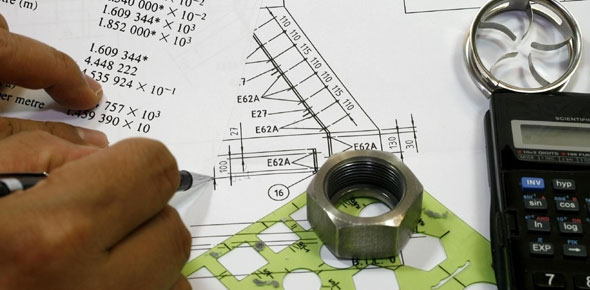# Mechanical Engineering Knowledge

6 Questions | Total Attempts: 5919SettingsThis quiz contain some questions about fundamentals in mechanical engineering. Most probably no one like the topic, but remember, this is just to test the website itself, rather than your knowledge

Related Topics
• 1.
What state the first law of Thermodynamics?
• A.

Conservation of Energy

• B.

Conservation of Mass

• C.

Conservation of Momentum

• D.

Conservation of Charge

• 2.
What states the second law of Thermodynamics?
• A.

All the heat entering in a system cannot be transformed to work

• B.

Entropy is always positive

• C.

Conservation of Charge

• D.

Force equilibrium

• 3.
How many degrees of freedom did a four bar linkage posses?
• A.

1

• B.

2

• C.

3

• D.

4

• 4.
Steel is an alloy between iron and...?
• A.

Copper

• B.

Bronze

• C.

Carbon

• D.

Chromium

• E.

Silver

• 5.
In a simple spring-mass system, stability with oscillations is obtained when the differential equation laplace transform have..?
• A.

Positive roots

• B.

Negative roots

• C.

Imaginary positive roots

• D.

Imaginary negative roots

• 6.
What describes the Navier Stokes equations?
• A.

Conduction heat transfer behavior

• B.

Fluid mechanics behavior

• C.

Combustion inside a piston-cylinder assembly

• D.

Rotary Machinery